We are working to support a site-wide PDF but it is not yet available. You can download PDFs for individual lectures through the download badge on each lecture page.

Code should execute sequentially if run in a Jupyter notebook

Optimal Taxation in an LQ Economy¶

Contents¶

In addition to what’s in Anaconda, this lecture will need the following libraries

In :
!pip install quantecon


Overview¶

In this lecture we study optimal fiscal policy in a linear quadratic setting

We slightly modify a well-known model of Robert Lucas and Nancy Stokey [LS83] so that convenient formulas for solving linear-quadratic models can be applied to simplify the calculations

The economy consists of a representative household and a benevolent government

The government finances an exogenous stream of government purchases with state-contingent loans and a linear tax on labor income

A linear tax is sometimes called a flat-rate tax

The household maximizes utility by choosing paths for consumption and labor, taking prices and the government’s tax rate and borrowing plans as given

Maximum attainable utility for the household depends on the government’s tax and borrowing plans

The Ramsey problem [Ram27] is to choose tax and borrowing plans that maximize the household’s welfare, taking the household’s optimizing behavior as given

There is a large number of competitive equilibria indexed by different government fiscal policies

The Ramsey planner chooses the best competitive equilibrium

We want to study the dynamics of tax rates, tax revenues, government debt under a Ramsey plan

Because the Lucas and Stokey model features state-contingent government debt, the government debt dynamics differ substantially from those in a model of Robert Barro [Bar79]

The treatment given here closely follows this manuscript, prepared by Thomas J. Sargent and Francois R. Velde

We cover only the key features of the problem in this lecture, leaving you to refer to that source for additional results and intuition

Model Features¶

• Representative household
• Stochastic dynamic programming over an infinite horizon
• Distortionary taxation

The Ramsey Problem¶

We begin by outlining the key assumptions regarding technology, households and the government sector

Technology¶

Labor can be converted one-for-one into a single, non-storable consumption good

In the usual spirit of the LQ model, the amount of labor supplied in each period is unrestricted

This is unrealistic, but helpful when it comes to solving the model

Realistic labor supply can be induced by suitable parameter values

Households¶

Consider a representative household who chooses a path $\{\ell_t, c_t\}$ for labor and consumption to maximize

$$-\mathbb E \frac{1}{2} \sum_{t=0}^{\infty} \beta^t \left[ (c_t - b_t)^2 + \ell_t^2 \right] \tag{1}$$

subject to the budget constraint

$$\mathbb E \sum_{t=0}^{\infty} \beta^t p^0_t \left[ d_t + (1 - \tau_t) \ell_t + s_t - c_t \right] = 0 \tag{2}$$

Here

• $\beta$ is a discount factor in $(0, 1)$
• $p_t^0$ is a scaled Arrow-Debreu price at time $0$ of history contingent goods at time $t+j$
• $b_t$ is a stochastic preference parameter
• $d_t$ is an endowment process
• $\tau_t$ is a flat tax rate on labor income
• $s_t$ is a promised time-$t$ coupon payment on debt issued by the government

The scaled Arrow-Debreu price $p^0_t$ is related to the unscaled Arrow-Debreu price as follows.

If we let $\pi^0_t(x^t)$ denote the probability (density) of a history $x^t = [x_t, x_{t-1}, \ldots, x_0]$ of the state $x^t$, then the Arrow-Debreu time $0$ price of a claim on one unit of consumption at date $t$, history $x^t$ would be

$$\frac{\beta^t p^0_t} {\pi_t^0(x^t)}$$

Thus, our scaled Arrow-Debreu price is the ordinary Arrow-Debreu price multiplied by the discount factor $\beta^t$ and divided by an appropriate probability

The budget constraint (2) requires that the present value of consumption be restricted to equal the present value of endowments, labor income and coupon payments on bond holdings

Government¶

The government imposes a linear tax on labor income, fully committing to a stochastic path of tax rates at time zero

The government also issues state-contingent debt

Given government tax and borrowing plans, we can construct a competitive equilibrium with distorting government taxes

Among all such competitive equilibria, the Ramsey plan is the one that maximizes the welfare of the representative consumer

Exogenous Variables¶

Endowments, government expenditure, the preference shock process $b_t$, and promised coupon payments on initial government debt $s_t$ are all exogenous, and given by

• $d_t = S_d x_t$
• $g_t = S_g x_t$
• $b_t = S_b x_t$
• $s_t = S_s x_t$

The matrices $S_d, S_g, S_b, S_s$ are primitives and $\{x_t\}$ is an exogenous stochastic process taking values in $\mathbb R^k$

We consider two specifications for $\{x_t\}$

1. Discrete case: $\{x_t\}$ is a discrete state Markov chain with transition matrix $P$
2. VAR case: $\{x_t\}$ obeys $x_{t+1} = A x_t + C w_{t+1}$ where $\{w_t\}$ is independent zero mean Gaussian with identify covariance matrix

Feasibility¶

The period-by-period feasibility restriction for this economy is

$$c_t + g_t = d_t + \ell_t \tag{3}$$

A labor-consumption process $\{\ell_t, c_t\}$ is called feasible if (3) holds for all $t$

Government budget constraint¶

Where $p_t^0$ is again a scaled Arrow-Debreu price, the time zero government budget constraint is

$$\mathbb E \sum_{t=0}^{\infty} \beta^t p^0_t (s_t + g_t - \tau_t \ell_t ) = 0 \tag{4}$$

Equilibrium¶

An equilibrium is a feasible allocation $\{\ell_t, c_t\}$, a sequence of prices $\{p_t^0\}$, and a tax system $\{\tau_t\}$ such that

1. The allocation $\{\ell_t, c_t\}$ is optimal for the household given $\{p_t^0\}$ and $\{\tau_t\}$
2. The government’s budget constraint (4) is satisfied

The Ramsey problem is to choose the equilibrium $\{\ell_t, c_t, \tau_t, p_t^0\}$ that maximizes the household’s welfare

If $\{\ell_t, c_t, \tau_t, p_t^0\}$ solves the Ramsey problem, then $\{\tau_t\}$ is called the Ramsey plan

The solution procedure we adopt is

1. Use the first-order conditions from the household problem to pin down prices and allocations given $\{\tau_t\}$
2. Use these expressions to rewrite the government budget constraint (4) in terms of exogenous variables and allocations
3. Maximize the household’s objective function (1) subject to the constraint constructed in step 2 and the feasibility constraint (3)

The solution to this maximization problem pins down all quantities of interest

Solution¶

Step one is to obtain the first-conditions for the household’s problem, taking taxes and prices as given

Letting $\mu$ be the Lagrange multiplier on (2), the first-order conditions are $p_t^0 = (c_t - b_t) / \mu$ and $\ell_t = (c_t - b_t) (1 - \tau_t)$

Rearranging and normalizing at $\mu = b_0 - c_0$, we can write these conditions as

$$p_t^0 = \frac{b_t - c_t}{b_0 - c_0} \quad \text{and} \quad \tau_t = 1 - \frac{\ell_t}{b_t - c_t} \tag{5}$$

Substituting (5) into the government’s budget constraint (4) yields

$$\mathbb E \sum_{t=0}^{\infty} \beta^t \left[ (b_t - c_t)(s_t + g_t - \ell_t) + \ell_t^2 \right] = 0 \tag{6}$$

The Ramsey problem now amounts to maximizing (1) subject to (6) and (3)

The associated Lagrangian is

$$\mathscr L = \mathbb E \sum_{t=0}^{\infty} \beta^t \left\{ -\frac{1}{2} \left[ (c_t - b_t)^2 + \ell_t^2 \right] + \lambda \left[ (b_t - c_t)(\ell_t - s_t - g_t) - \ell_t^2 \right] + \mu_t [d_t + \ell_t - c_t - g_t] \right\} \tag{7}$$

The first order conditions associated with $c_t$ and $\ell_t$ are

$$-(c_t - b_t ) + \lambda [- \ell_t + (g_t + s_t )] = \mu_t$$

and

$$\ell_t - \lambda [(b_t - c_t) - 2 \ell_t ] = \mu_t$$

Combining these last two equalities with (3) and working through the algebra, one can show that

$$\ell_t = \bar \ell_t - \nu m_t \quad \text{and} \quad c_t = \bar c_t - \nu m_t \tag{8}$$

where

• $\nu := \lambda / (1 + 2 \lambda)$
• $\bar \ell_t := (b_t - d_t + g_t) / 2$
• $\bar c_t := (b_t + d_t - g_t) / 2$
• $m_t := (b_t - d_t - s_t ) / 2$

Apart from $\nu$, all of these quantities are expressed in terms of exogenous variables

To solve for $\nu$, we can use the government’s budget constraint again

The term inside the brackets in (6) is $(b_t - c_t)(s_t + g_t) - (b_t - c_t) \ell_t + \ell_t^2$

Using (8), the definitions above and the fact that $\bar \ell = b - \bar c$, this term can be rewritten as

$$(b_t - \bar c_t) (g_t + s_t ) + 2 m_t^2 ( \nu^2 - \nu)$$

Reinserting into (6), we get

$$\mathbb E \left\{ \sum_{t=0}^{\infty} \beta^t (b_t - \bar c_t) (g_t + s_t ) \right\} + ( \nu^2 - \nu) \mathbb E \left\{ \sum_{t=0}^{\infty} \beta^t 2 m_t^2 \right\} = 0 \tag{9}$$

Although it might not be clear yet, we are nearly there because:

• The two expectations terms in (9) can be solved for in terms of model primitives
• This in turn allows us to solve for the Lagrange multiplier $\nu$
• With $\nu$ in hand, we can go back and solve for the allocations via (8)
• Once we have the allocations, prices and the tax system can be derived from (5)

Let’s consider how to obtain the term $\nu$ in (9)

If we can compute the two expected geometric sums

$$b_0 := \mathbb E \left\{ \sum_{t=0}^{\infty} \beta^t (b_t - \bar c_t) (g_t + s_t ) \right\} \quad \text{and} \quad a_0 := \mathbb E \left\{ \sum_{t=0}^{\infty} \beta^t 2 m_t^2 \right\} \tag{10}$$

then the problem reduces to solving

$$b_0 + a_0 (\nu^2 - \nu) = 0$$

for $\nu$

Provided that $4 b_0 < a_0$, there is a unique solution $\nu \in (0, 1/2)$, and a unique corresponding $\lambda > 0$

Let’s work out how to compute mathematical expectations in (10)

For the first one, the random variable $(b_t - \bar c_t) (g_t + s_t )$ inside the summation can be expressed as

$$\frac{1}{2} x_t' (S_b - S_d + S_g)' (S_g + S_s) x_t$$

For the second expectation in (10), the random variable $2 m_t^2$ can be written as

$$\frac{1}{2} x_t' (S_b - S_d - S_s)' (S_b - S_d - S_s) x_t$$

It follows that both objects of interest are special cases of the expression

$$q(x_0) = \mathbb E \sum_{t=0}^{\infty} \beta^t x_t' H x_t \tag{11}$$

where $H$ is a matrix conformable to $x_t$ and $x_t'$ is the transpose of column vector $x_t$

Suppose first that $\{x_t\}$ is the Gaussian VAR described above

In this case, the formula for computing $q(x_0)$ is known to be $q(x_0) = x_0' Q x_0 + v$, where

• $Q$ is the solution to $Q = H + \beta A' Q A$, and
• $v = \text{trace} \, (C' Q C) \beta / (1 - \beta)$

The first equation is known as a discrete Lyapunov equation, and can be solved using this function

Finite state Markov case¶

Next suppose that $\{x_t\}$ is the discrete Markov process described above

Suppose further that each $x_t$ takes values in the state space $\{x^1, \ldots, x^N\} \subset \mathbb R^k$

Let $h \colon \mathbb R^k \to \mathbb R$ be a given function, and suppose that we wish to evaluate

$$q(x_0) = \mathbb E \sum_{t=0}^{\infty} \beta^t h(x_t) \quad \text{given} \quad x_0 = x^j$$

For example, in the discussion above, $h(x_t) = x_t' H x_t$

It is legitimate to pass the expectation through the sum, leading to

$$q(x_0) = \sum_{t=0}^{\infty} \beta^t (P^t h)[j] \tag{12}$$

Here

• $P^t$ is the $t$-th power of the transition matrix $P$
• $h$ is, with some abuse of notation, the vector $(h(x^1), \ldots, h(x^N))$
• $(P^t h)[j]$ indicates the $j$-th element of $P^t h$

It can be show that (12) is in fact equal to the $j$-th element of the vector $(I - \beta P)^{-1} h$

This last fact is applied in the calculations below

Other Variables¶

We are interested in tracking several other variables besides the ones described above.

To prepare the way for this, we define

$$p^t_{t+j} = \frac{b_{t+j}- c_{t+j}}{b_t - c_t}$$

as the scaled Arrow-Debreu time $t$ price of a history contingent claim on one unit of consumption at time $t+j$

These are prices that would prevail at time $t$ if market were reopened at time $t$

These prices are constituents of the present value of government obligations outstanding at time $t$, which can be expressed as

$$B_t := \mathbb E_t \sum_{j=0}^{\infty} \beta^j p^t_{t+j} (\tau_{t+j} \ell_{t+j} - g_{t+j}) \tag{13}$$

Using our expression for prices and the Ramsey plan, we can also write $B_t$ as

$$B_t = \mathbb E_t \sum_{j=0}^{\infty} \beta^j \frac{ (b_{t+j} - c_{t+j})(\ell_{t+j} - g_{t+j}) - \ell^2_{t+j} } { b_t - c_t }$$

This version is more convenient for computation

Using the equation

$$p^t_{t+j} = p^t_{t+1} p^{t+1}_{t+j}$$

it is possible to verity that (13) implies that

$$B_t = (\tau_t \ell_t - g_t) + E_t \sum_{j=1}^\infty p^t_{t+j} (\tau_{t+j} \ell_{t+j} - g_{t+j})$$

and

$$B_t = (\tau_t \ell_t - g_t) + \beta E_t p^t_{t+1} B_{t+1} \tag{14}$$

Define

$$R^{-1}_{t} := \mathbb E_t \beta^j p^t_{t+1} \tag{15}$$

$R_{t}$ is the gross $1$-period risk-free rate for loans between $t$ and $t+1$

A Martingale¶

We now want to study the following two objects, namely,

$$\pi_{t+1} := B_{t+1} - R_t [B_t - (\tau_t \ell_t - g_t)]$$

and the cumulation of $\pi_t$

$$\Pi_t := \sum_{s=0}^t \pi_t$$

The term $\pi_{t+1}$ is the difference between two quantities:

• $B_{t+1}$, the value of government debt at the start of period $t+1$
• $R_t [B_t + g_t - \tau_t ]$, which is what the government would have owed at the beginning of period $t+1$ if it had simply borrowed at the one-period risk-free rate rather than selling state-contingent securities

Thus, $\pi_{t+1}$ is the excess payout on the actual portfolio of state contingent government debt relative to an alternative portfolio sufficient to finance $B_t + g_t - \tau_t \ell_t$ and consisting entirely of risk-free one-period bonds

Use expressions (14) and (15) to obtain

$$\pi_{t+1} = B_{t+1} - \frac{1}{\beta E_t p^t_{t+1}} \left[\beta E_t p^t_{t+1} B_{t+1} \right]$$

or

$$\pi_{t+1} = B_{t+1} - \tilde E_t B_{t+1} \tag{16}$$

where $\tilde E_t$ is the conditional mathematical expectation taken with respect to a one-step transition density that has been formed by multiplying the original transition density with the likelihood ratio

$$m^t_{t+1} = \frac{p^t_{t+1}}{E_t p^t_{t+1}}$$

It follows from equation (16) that

$$\tilde E_t \pi_{t+1} = \tilde E_t B_{t+1} - \tilde E_t B_{t+1} = 0$$

which asserts that $\{\pi_{t+1}\}$ is a martingale difference sequence under the distorted probability measure, and that $\{\Pi_t\}$ is a martingale under the distorted probability measure

In the tax-smoothing model of Robert Barro [Bar79], government debt is a random walk

In the current model, government debt $\{B_t\}$ is not a random walk, but the excess payoff $\{\Pi_t\}$ on it is

Implementation¶

The following code provides functions for

1. Solving for the Ramsey plan given a specification of the economy
2. Simulating the dynamics of the major variables

Description and clarifications are given below

In :
import sys
import numpy as np
from numpy import sqrt, eye, zeros, cumsum
from numpy.random import randn
import scipy.linalg
import matplotlib.pyplot as plt
from collections import namedtuple
from quantecon import nullspace, mc_sample_path, var_quadratic_sum

# == Set up a namedtuple to store data on the model economy == #
Economy = namedtuple('economy',
('β',          # Discount factor
'Sg',         # Govt spending selector matrix
'Sd',         # Exogenous endowment selector matrix
'Sb',         # Utility parameter selector matrix
'Ss',         # Coupon payments selector matrix
'discrete',   # Discrete or continuous -- boolean
'proc'))      # Stochastic process parameters

# == Set up a namedtuple to store return values for compute_paths() == #
Path = namedtuple('path',
('g',            # Govt spending
'd',            # Endowment
'b',            # Utility shift parameter
's',            # Coupon payment on existing debt
'c',            # Consumption
'l',            # Labor
'p',            # Price
'τ',            # Tax rate
'rvn',          # Revenue
'B',            # Govt debt
'R',            # Risk free gross return
'π',            # One-period risk-free interest rate
'Π',            # Cumulative rate of return, adjusted
'ξ'))           # Adjustment factor for Π

def compute_paths(T, econ):
"""
Compute simulated time paths for exogenous and endogenous variables.

Parameters
===========
T: int
Length of the simulation

econ: a namedtuple of type 'Economy', containing
β          - Discount factor
Sg         - Govt spending selector matrix
Sd         - Exogenous endowment selector matrix
Sb         - Utility parameter selector matrix
Ss         - Coupon payments selector matrix
discrete   - Discrete exogenous process (True or False)
proc       - Stochastic process parameters

Returns
========
path: a namedtuple of type 'Path', containing
g            - Govt spending
d            - Endowment
b            - Utility shift parameter
s            - Coupon payment on existing debt
c            - Consumption
l            - Labor
p            - Price
τ            - Tax rate
rvn          - Revenue
B            - Govt debt
R            - Risk free gross return
π            - One-period risk-free interest rate
Π            - Cumulative rate of return, adjusted
ξ            - Adjustment factor for Π

The corresponding values are flat numpy ndarrays.

"""

# == Simplify names == #
β, Sg, Sd, Sb, Ss = econ.β, econ.Sg, econ.Sd, econ.Sb, econ.Ss

if econ.discrete:
P, x_vals = econ.proc
else:
A, C = econ.proc

# == Simulate the exogenous process x == #
if econ.discrete:
state = mc_sample_path(P, init=0, sample_size=T)
x = x_vals[:, state]
else:
# == Generate an initial condition x0 satisfying x0 = A x0 == #
nx, nx = A.shape
x0 = nullspace((eye(nx) - A))
x0 = -x0 if (x0[nx-1] < 0) else x0
x0 = x0 / x0[nx-1]

# == Generate a time series x of length T starting from x0 == #
nx, nw = C.shape
x = zeros((nx, T))
w = randn(nw, T)
x[:, 0] = x0.T
for t in range(1, T):
x[:, t] = A @ x[:, t-1] + C @ w[:, t]

# == Compute exogenous variable sequences == #
g, d, b, s = ((S @ x).flatten() for S in (Sg, Sd, Sb, Ss))

# == Solve for Lagrange multiplier in the govt budget constraint == #
# In fact we solve for ν = lambda / (1 + 2*lambda).  Here ν is the
# solution to a quadratic equation a(ν**2 - ν) + b = 0 where
# a and b are expected discounted sums of quadratic forms of the state.
Sm = Sb - Sd - Ss
# == Compute a and b == #
if econ.discrete:
ns = P.shape
F = scipy.linalg.inv(eye(ns) - β * P)
a0 = 0.5 * (F @ (x_vals.T @ Sm.T)**2)
H = ((Sb - Sd + Sg) @ x_vals) * ((Sg - Ss) @ x_vals)
b0 = 0.5 * (F @ H.T)
a0, b0 = float(a0), float(b0)
else:
H = Sm.T @ Sm
a0 = 0.5 * var_quadratic_sum(A, C, H, β, x0)
H = (Sb - Sd + Sg).T @ (Sg + Ss)
b0 = 0.5 * var_quadratic_sum(A, C, H, β, x0)

# == Test that ν has a real solution before assigning == #
warning_msg = """
Hint: you probably set government spending too {}.  Elect a {}
Congress and start over.
"""
disc = a0**2 - 4 * a0 * b0
if disc >= 0:
ν = 0.5 * (a0 - sqrt(disc)) / a0
else:
print("There is no Ramsey equilibrium for these parameters.")
print(warning_msg.format('high', 'Republican'))
sys.exit(0)

# == Test that the Lagrange multiplier has the right sign == #
if ν * (0.5 - ν) < 0:
print("Negative multiplier on the government budget constraint.")
print(warning_msg.format('low', 'Democratic'))
sys.exit(0)

# == Solve for the allocation given ν and x == #
Sc = 0.5 * (Sb + Sd - Sg - ν * Sm)
Sl = 0.5 * (Sb - Sd + Sg - ν * Sm)
c = (Sc @ x).flatten()
l = (Sl @ x).flatten()
p = ((Sb - Sc) @ x).flatten()  # Price without normalization
τ = 1 - l / (b - c)
rvn = l * τ

# == Compute remaining variables == #
if econ.discrete:
H = ((Sb - Sc) @ x_vals) * ((Sl - Sg) @ x_vals) - (Sl @ x_vals)**2
temp = (F @ H.T).flatten()
B = temp[state] / p
H = (P[state, :] @ x_vals.T @ (Sb - Sc).T).flatten()
R = p / (β * H)
temp = ((P[state, :] @ x_vals.T @ (Sb - Sc).T)).flatten()
ξ = p[1:] / temp[:T-1]
else:
H = Sl.T @ Sl - (Sb - Sc).T @ (Sl - Sg)
L = np.empty(T)
for t in range(T):
L[t] = var_quadratic_sum(A, C, H, β, x[:, t])
B = L / p
Rinv = (β * ((Sb - Sc) @ A @ x)).flatten() / p
R = 1 / Rinv
AF1 = (Sb - Sc) @ x[:, 1:]
AF2 = (Sb - Sc) @ A @ x[:, :T-1]
ξ = AF1 / AF2
ξ = ξ.flatten()

π = B[1:] - R[:T-1] * B[:T-1] - rvn[:T-1] + g[:T-1]
Π = cumsum(π * ξ)

# == Prepare return values == #
path = Path(g=g, d=d, b=b, s=s, c=c, l=l, p=p,
τ=τ, rvn=rvn, B=B, R=R, π=π, Π=Π, ξ=ξ)

return path

def gen_fig_1(path):
"""
The parameter is the path namedtuple returned by compute_paths().  See
the docstring of that function for details.
"""

T = len(path.c)

# == Prepare axes == #
num_rows, num_cols = 2, 2
fig, axes = plt.subplots(num_rows, num_cols, figsize=(14, 10))
for i in range(num_rows):
for j in range(num_cols):
axes[i, j].grid()
axes[i, j].set_xlabel('Time')
bbox = (0., 1.02, 1., .102)
legend_args = {'bbox_to_anchor': bbox, 'loc': 3, 'mode': 'expand'}
p_args = {'lw': 2, 'alpha': 0.7}

# == Plot consumption, govt expenditure and revenue == #
ax = axes[0, 0]
ax.plot(path.rvn, label=r'$\tau_t \ell_t$', **p_args)
ax.plot(path.g, label='$g_t$', **p_args)
ax.plot(path.c, label='$c_t$', **p_args)
ax.legend(ncol=3, **legend_args)

# == Plot govt expenditure and debt == #
ax = axes[0, 1]
ax.plot(list(range(1, T+1)), path.rvn, label=r'$\tau_t \ell_t$', **p_args)
ax.plot(list(range(1, T+1)), path.g, label='$g_t$', **p_args)
ax.plot(list(range(1, T)), path.B[1:T], label='$B_{t+1}$', **p_args)
ax.legend(ncol=3, **legend_args)

# == Plot risk free return == #
ax = axes[1, 0]
ax.plot(list(range(1, T+1)), path.R - 1, label='$R_t - 1$', **p_args)
ax.legend(ncol=1, **legend_args)

# == Plot revenue, expenditure and risk free rate == #
ax = axes[1, 1]
ax.plot(list(range(1, T+1)), path.rvn, label=r'$\tau_t \ell_t$', **p_args)
ax.plot(list(range(1, T+1)), path.g, label='$g_t$', **p_args)
axes[1, 1].plot(list(range(1, T)), path.π, label=r'$\pi_{t+1}$', **p_args)
ax.legend(ncol=3, **legend_args)

plt.show()

def gen_fig_2(path):
"""
The parameter is the path namedtuple returned by compute_paths().  See
the docstring of that function for details.
"""

T = len(path.c)

# == Prepare axes == #
num_rows, num_cols = 2, 1
fig, axes = plt.subplots(num_rows, num_cols, figsize=(10, 10))
bbox = (0., 1.02, 1., .102)
bbox = (0., 1.02, 1., .102)
legend_args = {'bbox_to_anchor': bbox, 'loc': 3, 'mode': 'expand'}
p_args = {'lw': 2, 'alpha': 0.7}

# == Plot adjustment factor == #
ax = axes
ax.plot(list(range(2, T+1)), path.ξ, label=r'$\xi_t$', **p_args)
ax.grid()
ax.set_xlabel('Time')
ax.legend(ncol=1, **legend_args)

# == Plot adjusted cumulative return == #
ax = axes
ax.plot(list(range(2, T+1)), path.Π, label=r'$\Pi_t$', **p_args)
ax.grid()
ax.set_xlabel('Time')
ax.legend(ncol=1, **legend_args)

plt.show()


The function var_quadratic_sum imported from quadsums is for computing the value of (11) when the exogenous process $\{ x_t \}$ is of the VAR type described above

Below the definition of the function, you will see definitions of two namedtuple objects, Economy and Path

The first is used to collect all the parameters and primitives of a given LQ economy, while the second collects output of the computations

In Python, a namedtuple is a popular data type from the collections module of the standard library that replicates the functionality of a tuple, but also allows you to assign a name to each tuple element

These elements can then be references via dotted attribute notation — see for example the use of path in the functions gen_fig_1() and gen_fig_2()

The benefits of using namedtuples:

• Keeps content organized by meaning
• Helps reduce the number of global variables

Other than that, our code is long but relatively straightforward

Examples¶

Let’s look at two examples of usage

The Continuous Case¶

Our first example adopts the VAR specification described above

Regarding the primitives, we set

• $\beta = 1 / 1.05$
• $b_t = 2.135$ and $s_t = d_t = 0$ for all $t$

Government spending evolves according to

$$g_{t+1} - \mu_g = \rho (g_t - \mu_g) + C_g w_{g, t+1}$$

with $\rho = 0.7$, $\mu_g = 0.35$ and $C_g = \mu_g \sqrt{1 - \rho^2} / 10$

Here’s the code

In :
# == Parameters == #
β = 1 / 1.05
ρ, mg = .7, .35
A = eye(2)
A[0, :] = ρ, mg * (1-ρ)
C = np.zeros((2, 1))
C[0, 0] = np.sqrt(1 - ρ**2) * mg / 10
Sg = np.array((1, 0)).reshape(1, 2)
Sd = np.array((0, 0)).reshape(1, 2)
Sb = np.array((0, 2.135)).reshape(1, 2)
Ss = np.array((0, 0)).reshape(1, 2)

economy = Economy(β=β, Sg=Sg, Sd=Sd, Sb=Sb, Ss=Ss,
discrete=False, proc=(A, C))

T = 50
path = compute_paths(T, economy)
gen_fig_1(path)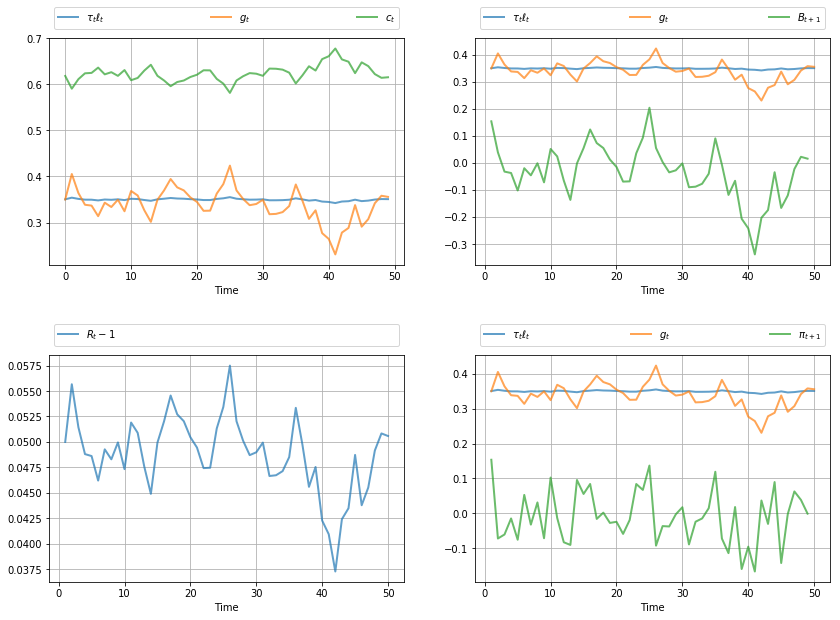The legends on the figures indicate the variables being tracked

Most obvious from the figure is tax smoothing in the sense that tax revenue is much less variable than government expenditure

In :
gen_fig_2(path)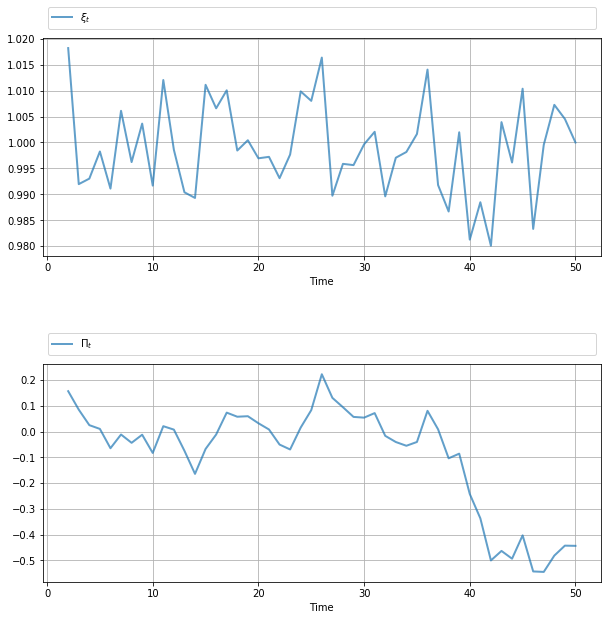See the original manuscript for comments and interpretation

The Discrete Case¶

Our second example adopts a discrete Markov specification for the exogenous process

In :
# == Parameters == #
β = 1 / 1.05
P = np.array([[0.8, 0.2, 0.0],
[0.0, 0.5, 0.5],
[0.0, 0.0, 1.0]])

# == Possible states of the world == #
# Each column is a state of the world. The rows are [g d b s 1]
x_vals = np.array([[0.5, 0.5, 0.25],
[0.0, 0.0,  0.0],
[2.2, 2.2,  2.2],
[0.0, 0.0,  0.0],
[1.0, 1.0,  1.0]])

Sg = np.array((1, 0, 0, 0, 0)).reshape(1, 5)
Sd = np.array((0, 1, 0, 0, 0)).reshape(1, 5)
Sb = np.array((0, 0, 1, 0, 0)).reshape(1, 5)
Ss = np.array((0, 0, 0, 1, 0)).reshape(1, 5)

economy = Economy(β=β, Sg=Sg, Sd=Sd, Sb=Sb, Ss=Ss,
discrete=True, proc=(P, x_vals))

T = 15
path = compute_paths(T, economy)
gen_fig_1(path)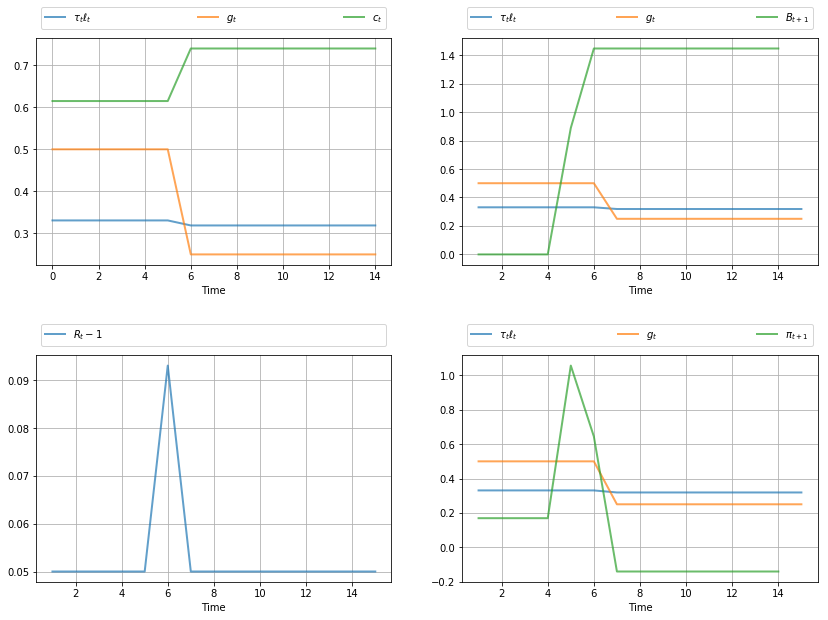The call gen_fig_2(path) generates

In :
gen_fig_2(path)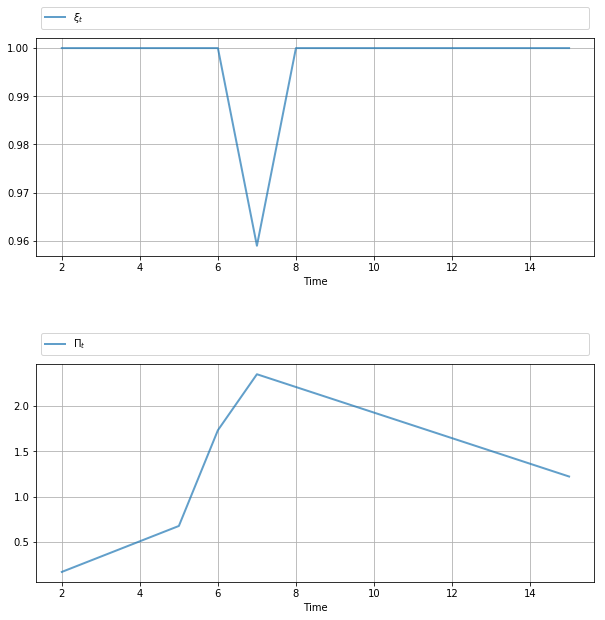See the original manuscript for comments and interpretation

Exercises¶

Exercise 1¶

Modify the VAR example given above, setting

$$g_{t+1} - \mu_g = \rho (g_{t-3} - \mu_g) + C_g w_{g, t+1}$$

with $\rho = 0.95$ and $C_g = 0.7 \sqrt{1 - \rho^2}$

Produce the corresponding figures

Solutions¶

Exercise 1¶

In :
# == Parameters == #
β = 1 / 1.05
ρ, mg = .95, .35
A = np.array([[0, 0, 0, ρ, mg*(1-ρ)],
[1, 0, 0, 0,        0],
[0, 1, 0, 0,        0],
[0, 0, 1, 0,        0],
[0, 0, 0, 0,        1]])
C = np.zeros((5, 1))
C[0, 0] = np.sqrt(1 - ρ**2) * mg / 8
Sg = np.array((1, 0, 0, 0, 0)).reshape(1, 5)
Sd = np.array((0, 0, 0, 0, 0)).reshape(1, 5)
Sb = np.array((0, 0, 0, 0, 2.135)).reshape(1, 5)  # Chosen st. (Sc + Sg) * x0 = 1
Ss = np.array((0, 0, 0, 0, 0)).reshape(1, 5)

economy = Economy(β=β, Sg=Sg, Sd=Sd, Sb=Sb,
Ss=Ss, discrete=False, proc=(A, C))

T = 50
path = compute_paths(T, economy)

gen_fig_1(path)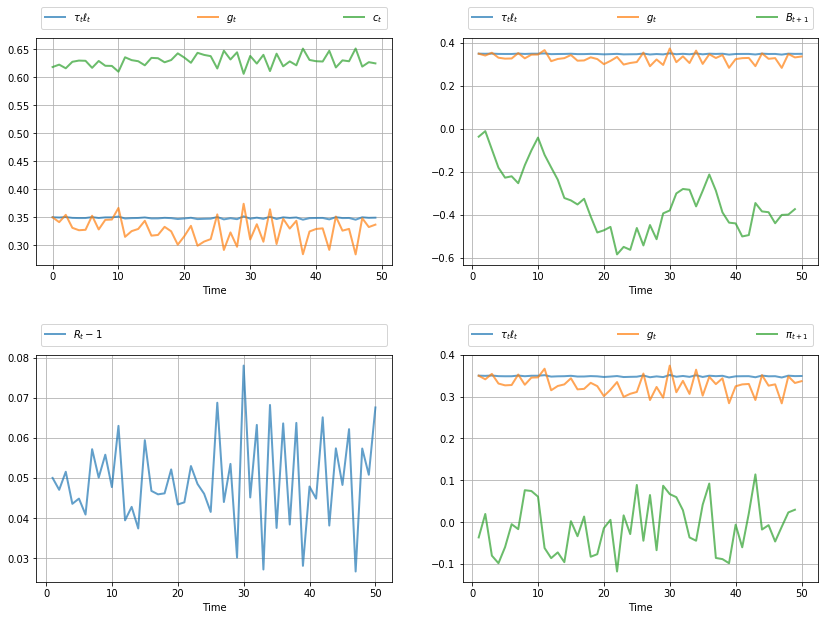In :
gen_fig_2(path)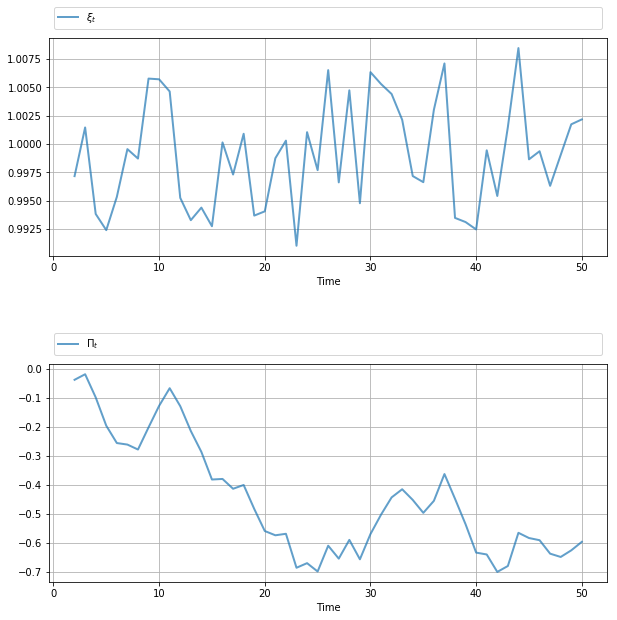• Share page